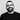# Some history about Functional programming - λ calculus!

April 11, 2018 , ☕️ 2 min read

### Some history about Functional programming - λ calculus

Functional Programming (FP) is not a new concept and it has some history that it based on to be at this form we use nowadays. this history starts with λ lambda calculus.

### λ lambda calculus

Lambda calculus was introduced by Alonzo church in the 1930s as apart of an investigation into the foundation mathematics.

Lambda calculus is a typeless theory of functions, it describe a notion of a function in computational perspective.

Function in lambda calculus is a black box , what it dose that it take some input, so may it takes a number like x and it’s going to process it in someway and it’s going to produce an output.

There’s two facts about functions in λ calculus:

• functions are black box so you are not allowed to look inside, you can’t see the mechanism of what’s going on.
• functions are pure so they have no internal state, no hidden information that we can use.

What’s the point of λ calculus?

• You can encode any computation into lambda calculus function.
• It build the basis of functional programming.

What you can do with λ calculus?

lambda calculus basically got nothing in it, no data types no recursion or even control structure, all we got is variables, a way of building functions and a way of applying those functions.

so we have to encode almost everything you need to build your program logical operators, loops, recursions, …etc

How to define functions in λ calculus?

To define a function that take single input x and increment that input by one you may define your function in that way

λx. x+1

x is the input, x+1 is the output

To define another function that take two inputs and return the summation as the output you may type your function in that way.

λx.λy x+y

here the function take two inputs x, y and return the summation of them as output.

also you can decode some logic operators as function in lambda calculus here an example, let’s encode true and false into functions

If true were a function it would be a function that take two arguments and return the first one,

so TRUEfunction would be like

λx.λy x

and the same for FALSE function it would be a function that takes two arguments and return the second one as output,

λx.λy y

That’s was a very simple introduction about lambda calculus.

Edit on GitHub